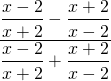Chapter 8: Rational Expressions

# 8.5 Reducing Complex Fractions

Complex fractions will have fractions in either the numerator, the denominator, or both. These fractions are generally simplified by multiplying the fractions in the numerator and denominator by the LCD. This process allows you to reduce a complex fraction to a simpler one in one step.

Example 8.5.1

Reduce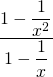.

For this fraction, the LCD is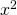. To simplify this complex fraction, multiply each term in the numerator and the denominator by the LCD.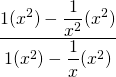This will result in: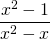Now, factor both the numerator and denominator, which results in: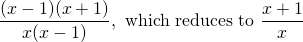It matters not how complex these fractions are: simply find the LCD to reduce the complex fraction to one that is simpler.

The more fractions there are in a problem, the more times the process is repeated.

Example 8.5.2

Reduce the following complex fraction: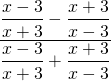For this fraction, the LCD is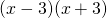. To simplify the above complex fraction, multiply both the numerator and denominator by the LCD. This looks like: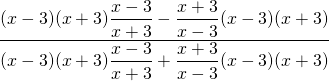Which reduces to: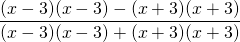Now multiply out the numerator and denominator and add like terms: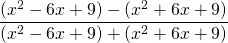Dropping the brackets leaves: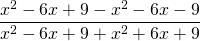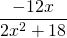You will notice there is a common factor of 2 in each of the terms that can be factored out. This results in: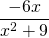# Questions

Simplify each of the following complex fractions.

1.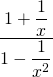2.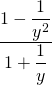3.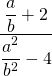4.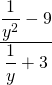5.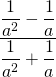6.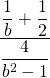7.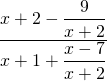8.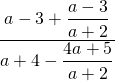9.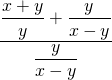10.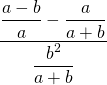11.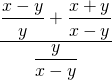12.